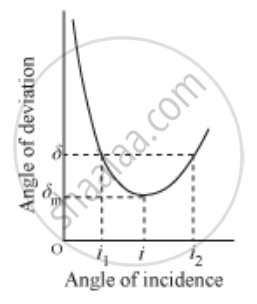# Plot a graph to show variation of the angle of deviation as a function of angle of incidence for light passing through a prism. Derive an expression for refractive index of the prism in terms of angle of minimum deviation and angle of prism. - Physics

Plot a graph to show the variation of the angle of deviation as a function of the angle of incidence for light passing through a prism. Derive an expression for the refractive index of the prism in terms of angle of minimum deviation and angle of the prism.

#### SolutionIf the angle of incidence is increased gradually, then the angle of deviation first decreases, attains a minimum value (δm) and then again starts increasing.

When the angle of deviation is minimum, the prism is said to be placed in the minimum deviation position.

There is only one angle of incidence for which the angle of deviation is minimum.

When δ = δm [prism in minimum deviation position],

e = i and r2 = r1 = r …..(i)

r1+r2=A

From (i), we get

r + r = A

r=A/2

Also, we have

A + δ = i + e

Substituting δ = δm and e = i,

A + δm = i + i

i=(A+delta_m)/2

mu=sin i/sin r

:.mu=(sin(A+delta_m/2)/Sin (A/2))

Concept: Dispersion by a Prism
Is there an error in this question or solution?
2015-2016 (March) Delhi Set 1

Share